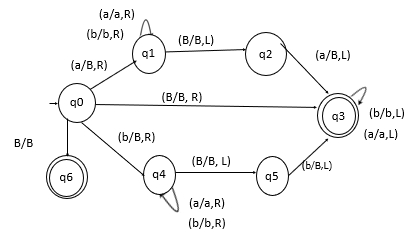# Construct a TM that accepts even-length palindromes over the alphabet {0,1}?

Data Structure AlgorithmsComputer ScienceComputers

A Turing machine (TM) is a 7-tuple (Q, ∑, Γ, δ, q0, qaccept , qreject).

Where,

• Q is a finite set of states.

• ∑ is the input alphabet that does not contain the blank symbol t.

• Γ is the tape alphabet, where t ∈ Γ and ∑ ⊆ Γ.

• δ: (Q × Γ) → (Q × Γ × {L, R}) is the transition function.

• q0 ∈ Q is the start state.

• qaccept ∈ Q is the accept state.

• qreject ∈ Q is the reject state, where qreject ≠ qaccept.

For accepting even-length palindrome over the alphabet {0,1}, follow the steps given below −

• Match the first and last element and erase them and go on doing the same. Once we reach epsilon without any mismatch then the string is even-length palindrome.

• For an even-length palindrome a TM is defined after the machine runs and erases the first and last element without encountering a mismatch. Later on the turing machine accepts the string and the string is even-length palindrome.

Let the string be −

110011

Then, we can encounter three cases, which are as follows −

Case 1 − If starting and ending matches and then erase the first and last one

After erase − 1001

Case 2 − If starting and ending matches and then erase the first and last one

After erase− 00

Case 3 − If starting and ending matches and then erase the first and last zero

After erase remains − epsilon

This is a Turing machine constructed which accepts an even-length palindrome.

The schematic diagram for TM accepting the even-palindrome is as follows −